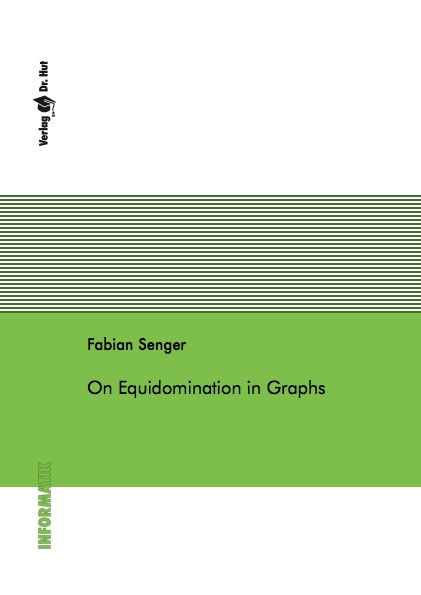Datenbestand vom 13. März 2019aktualisiert am 13. März 2019

# ISBN 9783843934893Euro 33,00 inkl. 7% MwSt

978-3-8439-3489-3, Reihe Informatik

Fabian Senger
On Equidomination in Graphs

123 Seiten, Dissertation Universität Köln (2017), Softcover, A5

## Zusammenfassung / Abstract

A graph G=(V,E) is called equidominating if there exists a value t in IN and a weight function w : V -> IN such that the total weight of a subset D of V is equal to t if and only if D is a minimal dominating set. Further, w is called an equidominating function, t a target value and the pair (w,t) an equidominating structure. To decide whether a given graph is equidominating is referred to as the EQUIDOMINATION problem.

First, we examine several results on standard graph classes and operations with respect to equidomination. Furthermore, we characterize hereditarily equidominating graphs. These are the graphs whose every induced subgraph is equidominating. For those graphs, we give a finite forbidden induced subgraph characterization and a structural decomposition. Using this decomposition, we state a polynomial time algorithm that recognizes hereditarily equidominating graphs.

We introduce two parameterized versions of the EQUIDOMINATION problem: the k-EQUIDOMINATION problem and the TARGET-t EQUIDOMINATION problem. For k in IN, a graph is called k-equidominating if we can identify the minimal dominating sets using only weights from 1 to k. In other words, if an equidominating function with co-domain {1,...,k} exists. For t in IN, a graph is said to be target-t equidominating if there is an equidominating structure with target value t.

For both parameterized problems we prove fixed-parameter tractability. The first step for this is to achieve the so-called pseudo class partition, which coarsens the twin partition. It is founded on the requirement that vertices from different blocks of the partition cannot have equal weights in any equidominating structure. Based on the pseudo class partition, we state an XP algorithm for the parameterized versions of the EQUIDOMINATION problem.

The second step is the examination of three reduction rules -- each of them concerning a specific type of block of the pseudo class partition -- which we use to construct problem kernels. The sizes of the kernels are bounded by a function depending only on the respective parameter. By applying the XP algorithm to the kernels, we achieve FPT algorithms.

The concept of equidomination was introduced nearly 40 years ago, but hardly any investigations exist. With this thesis, we want to fill that gap. We may lay the foundation for further research on equidomination.